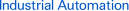Page top# input impedance

## Definition

The appropriate product category
The impedance as seen from the input terminal looking at the device when the device is operating. The input impedance is normally given as the equivalent value of a resistor and capacitor connected in parallel.
The appropriate product category
The impedance caused by the input circuit limiting resistor. With a constant-current input circuit, the impedance varies with the input voltage.
The appropriate product category
The impedance of an input to a device in operating status viewed from the input terminal. The input impedance is generally given as the equivalent resistance and capacitance connected in parallel. For DC measurement devices, simply the input resistance is given.